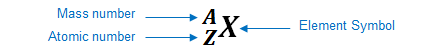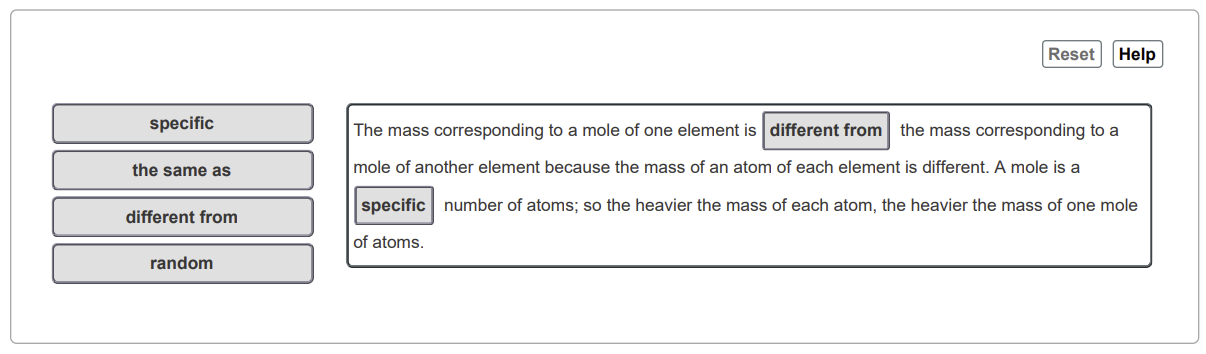# Problem: Why is the mass corresponding to a mole of one element different from the mass corresponding to a mole of another element?

###### FREE Expert Solution

We’re being asked to determine the name of the element that has an atomic number of 59.

An element can be represented as:You can determine the identity of the element using its atomic number:

Atomic number (Z):

84% (197 ratings)###### Problem Details

Why is the mass corresponding to a mole of one element different from the mass corresponding to a mole of another element?